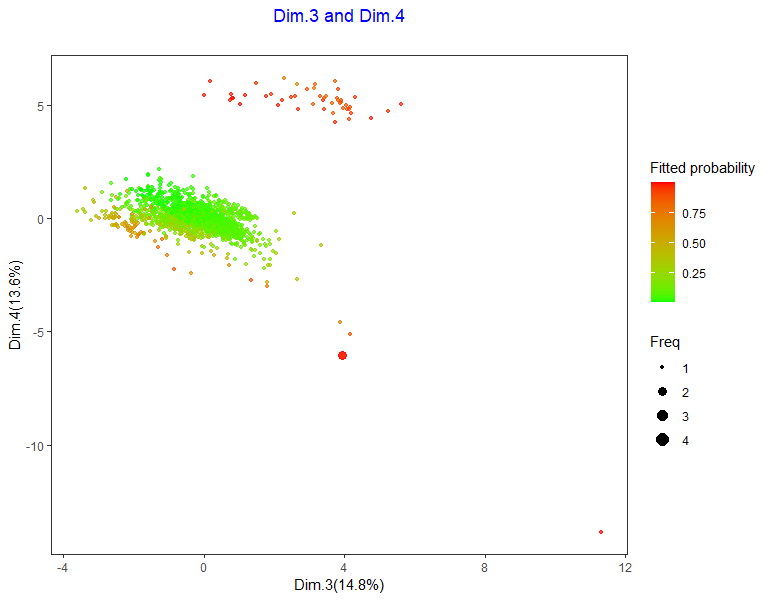# Using external predictions

External vector of probabilities can be used as base for contour and prediction plots. For example, one can use other algorithms, packages or other external sources to compute these probabilities and pass them through the 'proba' vector. Besides that, although basic function settings rely on fit and not on out-of-sample prediction, train and test predictions can also be represented.

In the next example, data is splitted into train and test. Test predictions are obtained with model built with train data, and then incorporated to famdcontour function through 'proba' vector. Plot for test points is shown.

If one wants to apply this procedure, caution is necessary:
1) Reduce dataset to input and vardep variables
2) verify the class predicted is the minority class within the predictive algorithm used
3) use selec=0 in famdcontour function to avoid further selection of variables
4) As algorithm need not to be applied in function famdcontour, use modelo=“glm”
5) Using an external vector of probabilities requires that the order of observations in probability vector coincides with the order in the dataset passed to the famdcontour function.

In the example, test data (same number of rows as proba) is passed to the famdcontour function.
This setting can be altered using cross validation, for example, with caret package, using all data.

``````library(visualpred)
dataf<-na.omit(Hmda)
listconti<-c("dir", "lvr", "ccs","uria")
listclass<-c("pbcr", "dmi", "self")
vardep<-c("deny")
dataf<-dataf[,c(listconti,listclass,vardep)]
set.seed(123)
train_ind <- sample(seq_len(nrow(Hmda)), size = 1700)
train <- dataf[train_ind, ]
test <- dataf[-train_ind, ]
formu<-paste("factor(",vardep,")~.")
proba<- predict(model,test,type="response")
result<-famdcontour(dataf=test,listconti=listconti,listclass=listclass,vardep=vardep,
proba=proba,title="Test Contour under GLM",title2="  ",selec=0,modelo="glm",classvar=0)

result[]
``````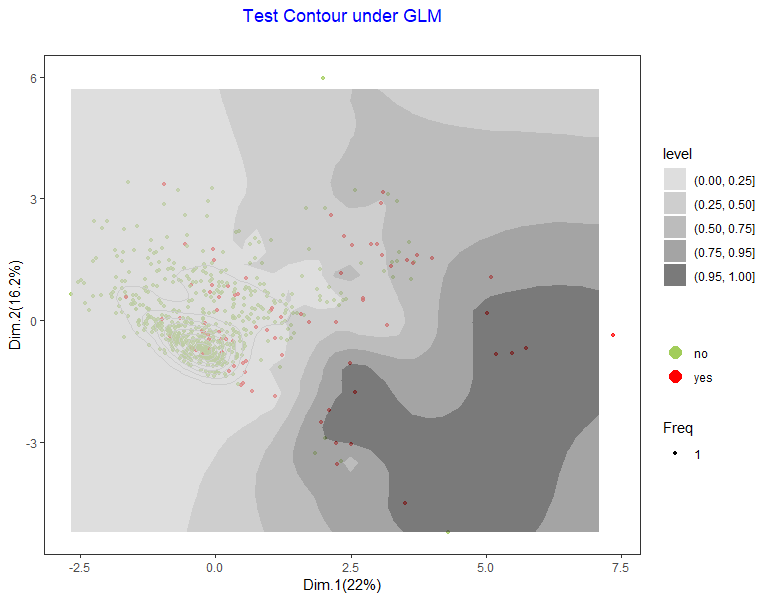``````library(randomForest)
model <- randomForest(formula(formu),data=train,mtry=4,nodesize=10,ntree=300)
proba <- predict(model, test,type="prob")
proba<-as.vector(proba[,2])

result<-famdcontour(dataf=test,listconti=listconti,listclass=listclass,vardep=vardep,
proba=proba,title="Test Contour under Random Forest",title2="  ",Dime1="Dim.1",Dime2="Dim.2",
selec=0,modelo="glm",classvar=0)

result[]
``````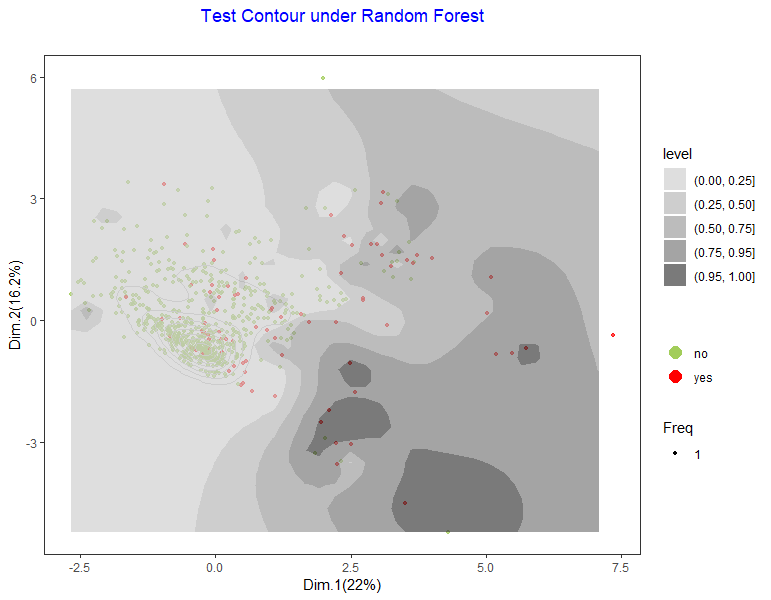# Colors and titles

Dependent variable colors can be set in a vector named depcol where the first color corresponds to the majority class. Color schema for variables and categories can also be set in a vector named listacol. In this example dependent variable colors are changed. Also, title schema can be overwritten with usual ggplot settings.

``````library(ggplot2)
library(visualpred)
dataf<-na.omit(Hmda)
listconti<-c("dir", "lvr", "ccs","uria")
listclass<-c("pbcr", "dmi", "self")
vardep<-c("deny")

result<-famdcontour(dataf=dataf,listconti=listconti,listclass=listclass,vardep=vardep,
title="",title2="  ",selec=0,modelo="glm",classvar=0,depcol=c("gold2","deeppink3"))

result[]+
ggtitle("Hmda data",subtitle="2380 obs")+theme(
plot.title = element_text(hjust=0.5,color="darkorchid"),
plot.subtitle= element_text(hjust=0.5,color="violet")
)
``````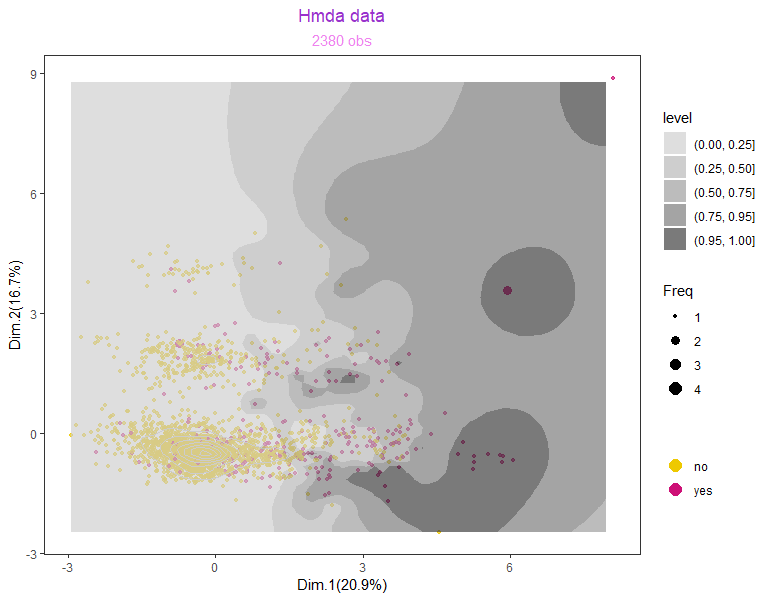# Plotting over selected dimensions

Plot over other Dimensions built by famd or mca algorithms is allowed, as in the next example.

``````result<-famdcontour(dataf=dataf,listconti=listconti,listclass=listclass,vardep=vardep,
title="Dim.1 and Dim.2",title2="  ",Dime1="Dim.1",Dime2="Dim.2",
selec=0,modelo="glm",classvar=0)

result[]
``````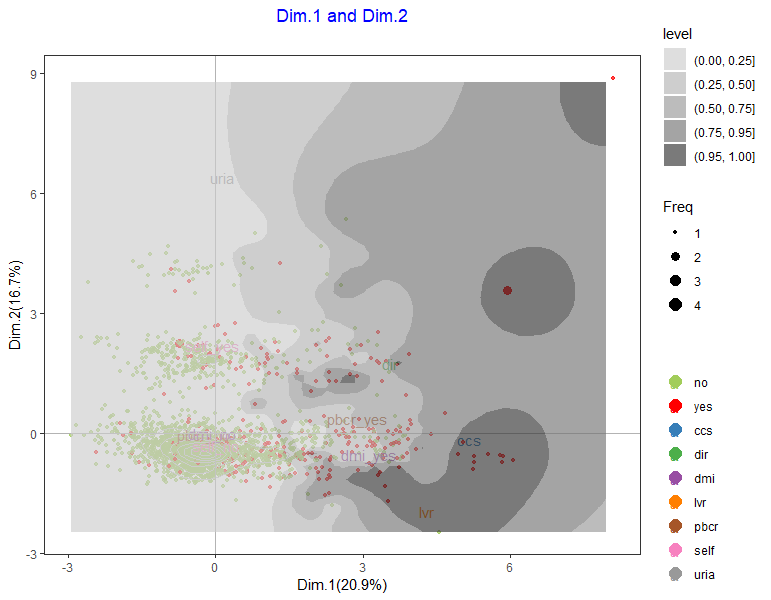``````result[]
``````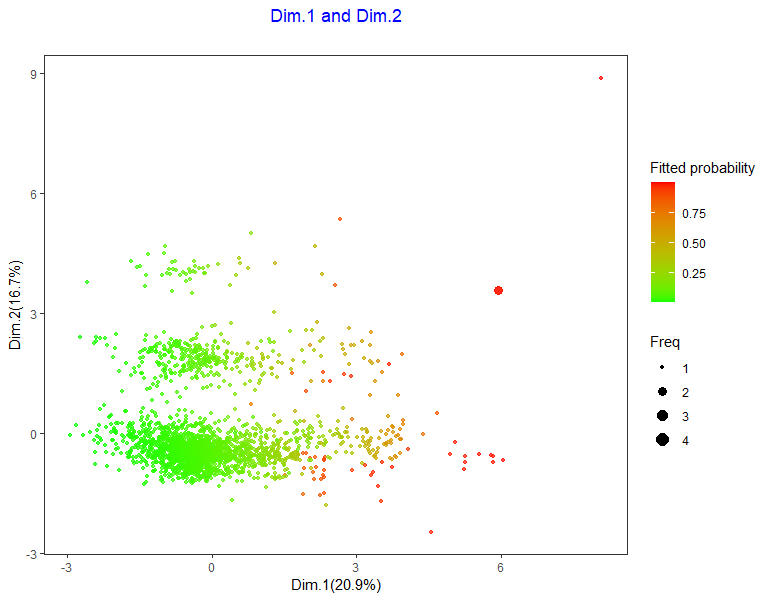``````result<-famdcontour(dataf=dataf,listconti=listconti,listclass=listclass,vardep=vardep,
title="Dim.3 and Dim.4",title2="  ",Dime1="Dim.3",Dime2="Dim.4",
selec=0,modelo="glm",classvar=0)

result[]
``````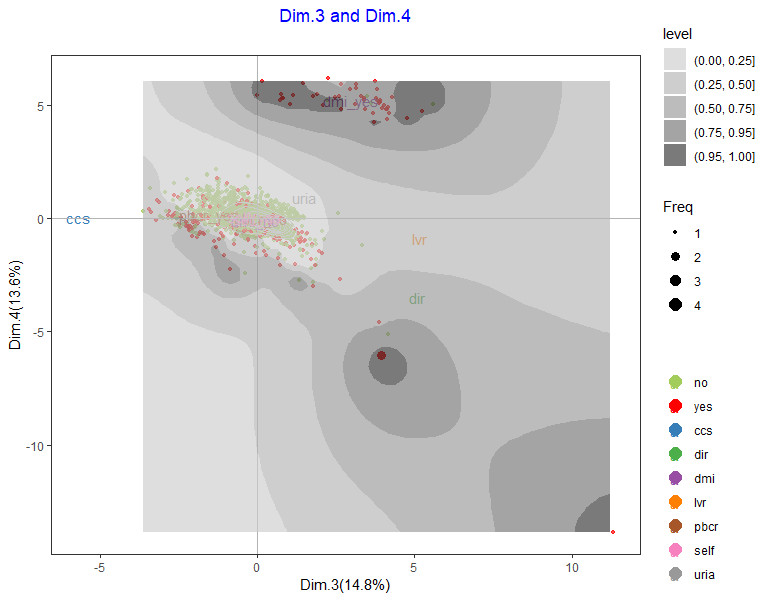``````result[]
``````